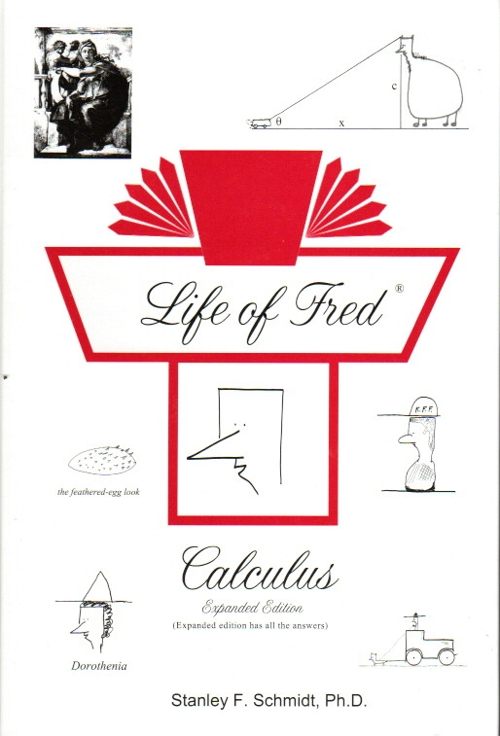Life of Fred Calculus \$57 : Functions, Limits, Speed, Slope, Derivatives, Concavity, Trig, Related Rates, Curvature, Integrals, Area, Work, Centroids, Logs, Conics, Infinite Series, Solids of Revolution, Polar Coordinates, Hyperbolic Trig, Vectors, Partial Derivatives, Double Integrals, Vector Calculus, Differential Equations. (Sixteen units of college calculus.) This book includes the standard advanced topics such as the epsilon-delta proofs, proof of the Mean Value Theorem, probability density functions, proof that an increasing bounded sequence has a finite limit, Legrange Multipliers, Cylindrical and Spherical Coordinate Systems, Green's Theorem, Divergence Theorem, Stoke's Theorem, six approaches to First Order Differential Equations (variables separable, homogeneous, first order linear, Bernoulli's equation, Exact, and 12 Integrating Factors), and two approaches to Second Order Differential Equations. ISBN: 978-0-9709995-53-1 hardback, 592 pages. \$57